# Using Deep Learning Techniques to Classify Devanagari Handwritten Digits | PyTorch Tutorial

In this article we'll define and train a simple convolutional neural network to recognize Devanagari Handwritten digits. This task is very similar to the famous MNIST character recognition problem which is commonly known as a "Hello World" problem for deep learning.

### Devanagari Script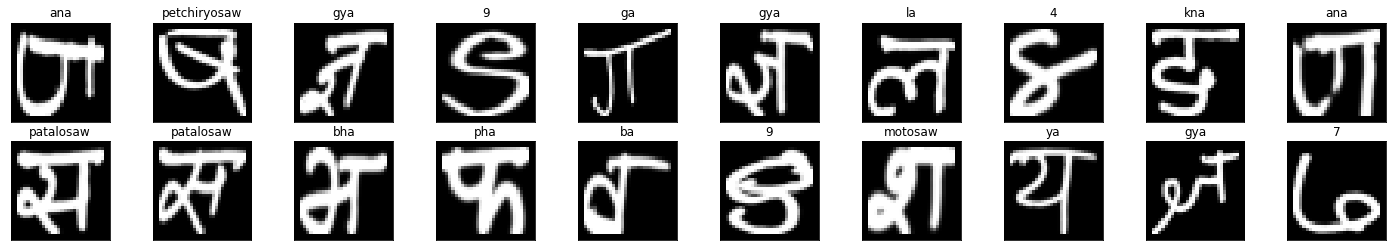Sample of a Devanagari Characters

Devanagari script is used in writing Sanskrit, Hindi, Marathi, Nepali and it's modified version is used in writing Bengali and Punjabi too. Devanagari is made up of two words "Deva" + "Nagari", "Deva" means gods and "Nagari" means city. Some say Devanagari came from the city of gods.

The Nepalese derivation of Devanagari consists of 36 constants, 12 vowels and 10 numeric characters.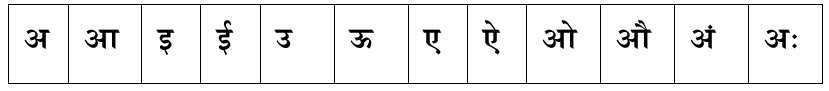Devanagari Vowels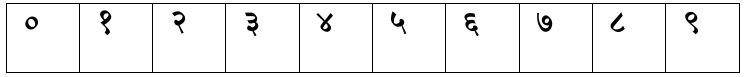Devanagari Numerals

### Convolutional Neural Network(CNN)

We will design a simple CNN to recognize a handwritten digits. In Deep Learning, A Convolutional Neural Network is a type of artificial neural network originally designed for image analysis.  They are often called ConvNet. CNN has deep feed-forward architecture and has unbelievably good generalizing capability than other networks with fully connected layers. Convolutional Neural Network

We will use DHCD dataset to train our neural network. DHCD details:

• Total Images: 92,000, Training(85%): 78,200 and Testing(15%): 13,800
• Each image is 32x32 pixels and the actual character is centered within 28x28 pixels.

We further divided the training set into training and validation set with the split of 90:10. If we desire, we can use the k-fold cross-validation scheme to train the model with entire 78,200 images instead of the 90%.

The model was trained with batch size of 16,32 and epoch 50, 70, 100 on all cases the average test accuracy was greater than 98%.

### Code

#### Import all necessary packages

First thing first, let's import all necessary packages. We will import torch, numpy, matplotlib and other important subpackages.

import torch
import torch.nn as nn
import numpy as np
import matplotlib.pyplot as plt
from torchvision import datasets, transforms
import torch.nn.functional as F
from torch.utils.data.sampler import SubsetRandomSampler
import torch.optim as optim

Data Augmentation

Our neural network is only as good the data we feed it. . Most popular datasets have millions of images and most popular neural net architecture are trained with more than a millions of images or videos. We certainly can't be upset and sit down, what we can do is augment the datasets.

We can randomly flip, rotate, introduce noise and apply a bunch of transforms so that our training set represent more general scenario than the data we have. By doing data augmentation we are increasing the capacity of our neural network to tune the parameters without increasing the data size.

Training, Validating and Testing

In this step, we will load the training and testing data. Further, we will divide the training data into validation and training sets. The validation set will be used to validate the hyperparameters we used and training set will be used to find the accuracy of the model. Right now, we are not going to use the K-fold cross validation, so choosing either 80:20 split or less than 20% for the validation set will be better.

Normalize ?

Normalize(mean=(0.5,), std=(0.5)) does the following for each channel:

image=imagemeanstd

With mean=0.5 and std-0.5, the image will be normalized the range [-1,1].

For example, the minimum value 0 will be converted to

00.50.5=1
, the maximum value of 1 will be converted to
10.50.5=1

To get our image back in [0,1] range, we could use,

image=((imagestd)+mean)

train_transform = transforms.Compose([
transforms.RandomRotation(10),
transforms.RandomAffine(degrees=45, translate=(0.1, 0.1), scale=(0.8, 1.2)),
transforms.RandomCrop(32),
transforms.ToTensor(),
transforms.Normalize((0.5, ), (0.5, ))
])

test_transform = transforms.Compose([
transforms.ToTensor(),
transforms.Normalize((0.5, ), (0.5, ))
])

Next, we will split the training set into training and validating set

batch_size = 32
valid_size = 0.10

num_train = len(train_data)
split_point = int(valid_size * num_train)

indices = list(range(num_train))
np.random.shuffle(indices)

valid_indices = indices[:split_point]
train_indices = indices[split_point:]

train_sampler = SubsetRandomSampler(train_indices)
valid_sampler = SubsetRandomSampler(valid_indices)

The loader combines a dataset and a sampler, and provides an iterable over the given dataset.

batch_size=batch_size, sampler=train_sampler)
batch_size=batch_size, sampler=valid_sampler)
batch_size=batch_size, shuffle=True)

#### Visualizing a batch of training data

train_on_gpu = torch.cuda.is_available()
# obtain one batch of test images
images, labels = dataiter.next()
images.numpy()

# move model inputs to cuda, if GPU available
if train_on_gpu:
images = images.cuda()

# plot the images in the batch, along with predicted labels
fig = plt.figure(figsize=(25, 4))
for idx in np.arange(20):
ax = fig.add_subplot(2, 20/2, idx+1, xticks=[], yticks=[])
img = images.cpu()[idx]
img = img * 0.5 + 0.5
img = np.transpose(img, (1, 2, 0))
plt.imshow(img)
ax.set_title(test_data.classes[labels[idx].item()])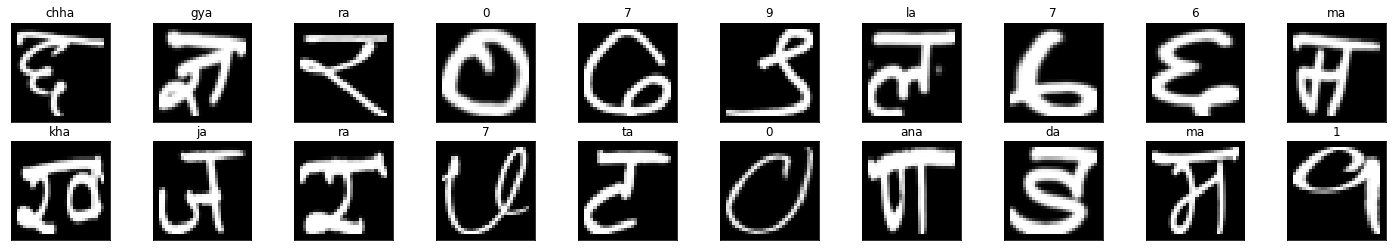Sample batch

### CNN Architecture

class Network(nn.Module):
def __init__(self):
super().__init__()
# First layer sees: 32x32x3
self.conv1 = nn.Conv2d(in_channels=3, out_channels=16,
# Second layer sees: 28x28x16
self.conv2 = nn.Conv2d(in_channels=16, out_channels=32,
# Third layer sees: 24x24x32
self.conv3 = nn.Conv2d(in_channels=32, out_channels=64,
# This layer output 20 x 20 x 64
self.fc1 = nn.Linear(20*20*64, 1000)
self.fc2 = nn.Linear(1000, output_size)
self.dropout = nn.Dropout(p=0.25)
def forward(self, x):
x = F.relu(self.conv1(x))
x = F.relu(self.conv2(x))
x = F.relu(self.conv3(x))
x = x.view(-1, 20*20*64)
x = self.dropout(x)
x = F.relu(self.fc1(x))
x = self.dropout(x)
x = self.fc2(x)
return x

Our CNN architecture is very simple. It takes input image of dimension 32x32x3, pass it through a convolution layer which give output 28x28x28 output vector. This process continues until the final layer outputs a 20x20x64 dimension vector. The image height and width are shrinking but the depth is increasing. Next we will flatten this 20x20x64 output vector and pass it through a linear layer. The output of linear layer goes through a dropout layer and finally it goes to another linear layer and a final output of 1000xoutput_size is generated. The output_size will be the number of classes.

Next, we will define our optimizer and loss function as:

criterion = nn.CrossEntropyLoss()
optimizer = optim.SGD(dhcd_model.parameters(), lr=0.001, momentum=0.9)

#### Training

n_epochs = 100
train_losses = []
valid_losses = []
valid_loss_min = np.inf

for e in range(n_epochs):
train_loss = 0
valid_loss = 0
dhcd_model.train()
if train_on_gpu:
img = img.cuda()
label = label.cuda()
predicted_label = dhcd_model(img)
loss = criterion(predicted_label, label)
loss.backward()
optimizer.step()
train_loss = train_loss + loss.item()
dhcd_model.eval()
if train_on_gpu:
img = img.cuda()
label = label.cuda()
predicted_label = dhcd_model(img)
loss = criterion(predicted_label, label)
valid_loss = valid_loss + loss.item()
train_losses.append(train_loss)
valid_losses.append(valid_loss)
print("Epoch: {} Train Loss: {} Valid Loss: {}".format(e+1,
train_loss, valid_loss))
if valid_loss < valid_loss_min:
print("Validation Loss Decreased From {} to {}".format(valid_loss_min,
valid_loss))
valid_loss_min = valid_loss
torch.save(dhcd_model.state_dict(), "dhcd_model_8_March_2020.pth")
print("Saving Best Model")

### Plotting Loss

fig, axes = plt.subplots(nrows=1, ncols=1)
axes.plot(train_losses, label="Training")
axes.plot(valid_losses, label="Validating")
axes.legend(frameon=False)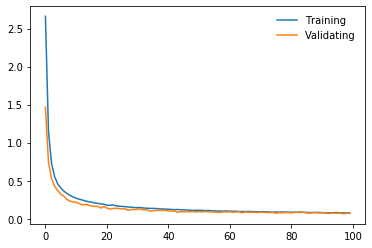Train and Valid Losses

### Testing the model

n_epochs = 50
avg_accuracy = 0
total_accuracy = 0
test_loss = 0
accuracy = 0
dhcd_model.eval()

for epoch in range(n_epochs):
if train_on_gpu:
img = img.cuda()
label = label.cuda()
predicted_label = dhcd_model(img)
loss = criterion(predicted_label, label)
test_loss = test_loss + loss.item()

top_probab, top_label = predicted_label.topk(1, dim=1)
equals = top_label == label.view(*top_label.shape)
accuracy = accuracy + torch.mean(equals.type(torch.FloatTensor))

total_accuracy = total_accuracy + accuracy

print("Epoch: {} Test Loss: {} Accuracy: {}".format(epoch+1,
test_loss, accuracy))

avg_accuracy = total_accuracy/(n_epochs) * 100
print("____\nAverage Accuracy: {:.3f}%\n____".format(avg_accuracy))

### Testing Model on a Sample Batch

# obtain one batch of test images
images, labels = dataiter.next()
images.numpy()

# move model inputs to cuda, if GPU available
if train_on_gpu:
images = images.cuda()

# get sample outputs
output = dhcd_model(images)
# convert output probabilities to predicted class
_, preds_tensor = torch.max(output, 1)
preds = np.squeeze(
preds_tensor.numpy()) if not train_on_gpu else np.squeeze(
preds_tensor.cpu().numpy())

# plot the images in the batch, along with predicted and true labels
fig = plt.figure(figsize=(25, 4))
for idx in np.arange(16):
ax = fig.add_subplot(2, 20/2, idx+1, xticks=[], yticks=[])
img = images.cpu()[idx]
img = img * 0.5 + 0.5
img = np.transpose(img, (1, 2, 0))
plt.imshow(img)
ax.set_title("{} ({})".format(train_data.classes[preds[idx].item()],
train_data.classes[labels[idx].item()]),
color=("green" if preds[idx]==labels[idx].item() else "red"))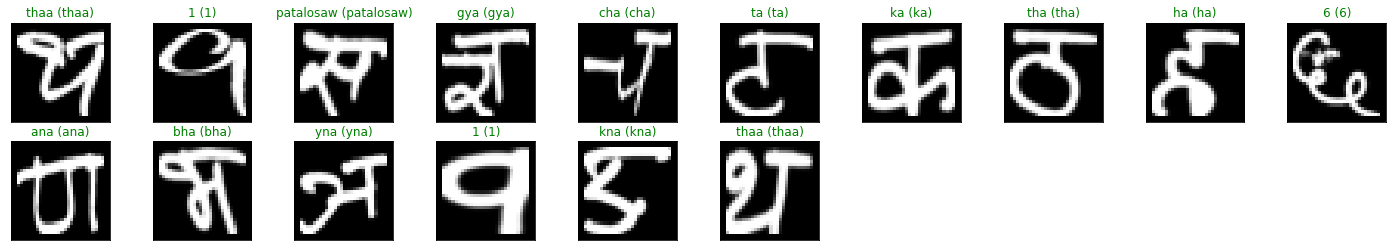Testing model on a Sample Batch

### Conclusion Remarks

We have seen that using a very simple CNN yeilds a very high accuracy. Here, we have just seen the basics of deep learning for Devanagari Characters. Follow us for more interesting tutorials and posts.

Cheers!!